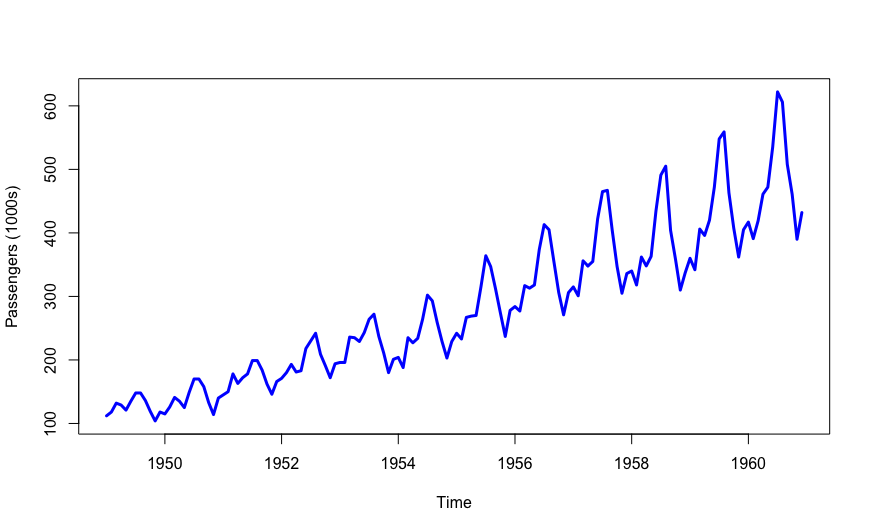# Decomposition methods for time series forecasting

## STATISTICAL FORECASTINGIf you have read the article Time series analysis for statistical forecasting, you already know that a time series is simply a sequence of values temporarily sorted. However, if such a sequence represents a real behaviour of something (for example the sales history of your business), it will be more than a simple sequence of values.

Therefore, a not trivial time series may be characterized by four different components:

• trend (T): represents the long term behaviour
• cycle (C): represents long term fluctuations due to external factors
• seasonality (S): represents repeating patterns in certain periods
• randomness (R): represents something which is not explicable

### How can we merge those components in one time series?

One of the most used formulation is the additive formulation. It says that the time series is simply a sum of the four components. Hence, if Y is our time series, this formulation says that Y = T+C+S+R.

This is a suitable solution when the seasonal component does not vary its intensity with the level of the time series (as in figure above).

Otherwise, a multiplicative formulation could be the solution. As expected, this formulation says that the time series is a multiplication of the four components, so Y = T*C*S*R.

### Methods for the estimation of the components

First of all, estimating the components of the time series is not trivial. There were several methods for extracting cycle-trend or seasonality components. For example, for extracting polynomial trend we can use a least squares approach. If the only trend affects the time series, we can write

Y = a0 + a1 * t + a2 * t2 + … + an * tn.

The a values could be estimated using a least squares approach. Suppose you have a monthly time series and you want to extract the seasonality. A possible way to do it, is to find a linear dependence between the current month and the previous ones. Hence, if you want to know the value for September 2017 the seasonal component might be

YSep 2017 = a0 + a1 * YSep 2016 + a2 YSep 2015.

In conclusion, altough the decomposition methods are not the state of art for statistical time series forecasting, they represent a milestone for the literature and cannot be ignored.

August 24, 2017 -

Need more info? Send us a message to know everything about intuendi.com